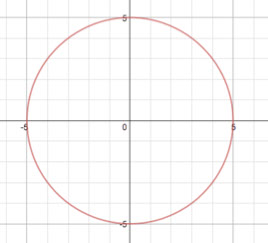# Parabolas Lesson 3 Episode 5 (Teachers)

### Reflecting

Sasha and Keoni share ideas about the usefulness of the equation
x = √(4y), which they derived in Episode 2.

### Episode SupportsStudents’ Conceptual Challenges

Students might not notice the connection between the general equation and the earlier procedure they used several times to solve for the x value for a known y value of a point on the parabola.

When asked to find the x value of when y is 50, Keoni writes down the equation that represents the Pythagorean theorem. Sasha jokes with him that he likes the “long way.” Sasha is prompted to clarify what she means by the long way. Together they solve for x using both procedures, the Pythagorean theorem equation and the general equation, x = √(4y). They notice that they get the same answers.

Focus Questions

For use in a classroom, pause the video and ask these questions:

1. [Pause video at 2:27]. Sasha says “you just like the long way.” What does she mean by the long way?

2. [Pause video at 4:26].What do you think? Will Sasha’s method get the same answer? Why or why not?

Supporting Dialogue

Build on students’ responses to the question: “what does Sasha mean by the long way?” by asking:

1. Kara, can you repeat what Dan said about the long way?

2. How about someone else? What do you think about what Keoni has written?

Math Extensions

The points on the circle below can be represented by equations. There are two possible representations in the table below.1. What is the difference between the two representations? Solve for the x-value when the y-value is 1.5.

2. What is meant by the symbol ±? How does it relate to the shape of the circle?